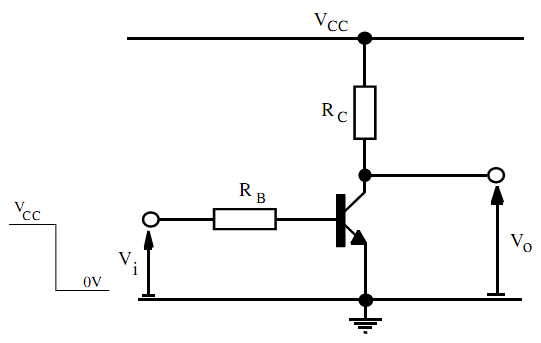By Charge Control equation determine collector current

In the individual transistor inverter circuit demonstrated below, the input voltage is switched abruptly by Vcc to 0V. There base current as acquired from the Charge Control equations governing turn-off of the transistor is specified as:

IB = (τFBF) iC + (τFBF) CBCRC (dic/dt) + τF(dic/dt) + CBCRC(dic/dt) = - VBE ON/RB

Determine this equation to acquire an expression for the collector current and the output voltage of the inverter throughout turn-off of the transistor. Therefore evaluate the 10 to 90 percent rise-time of the output voltage of the inverter when τF = 0.2ns, βF = 50, RC = 1kΩ, RB = 10kΩ and CBC = 0.5pF as well as VCC = 5V.#### Related Questions in Electrical Engineering

• ##### Q :Addition and multiplication of floating

Explain how the addition & multiplication can be carried out in the floating point arithmetic?

• ##### Q :SF6 Circuit Breaker and Ferranti effect

Define:

a) SF6 Circuit Breaker

b) Ferranti effect

• ##### Q :Scan design and generic forms of scan

Specify three generic forms of scan designs. Explain what is meant by the scan register?

• ##### Q :Basic Column Control please see the

please see the attachment. so, you can see figures. it helps you to write a report only 3 pages. write a short report that analyses the P&ID: i. A one-paragraph introduction. ii. A paragraph about the process. iii. The instruments that are used (describe their tags following the slides). iv.

• ##### Q :Explain Programmable Intelligent

What is a Programmable Intelligent Computer?

• ##### Q :Interrupts of 8051 micro controller

Explain various interrupts of the 8051 micro controller?

• ##### Q :What is Genetic algorithm Explain the

Explain the term Genetic algorithm. Describe it in brief.

• ##### Q :Illustrates the uses of timers

Illustrates the uses of timers?

• ##### Q :Determine the voltages at each of the

Sketch up a table demonstrating the states of conduction of each transistor within the TTL gate demonstrated below for all possible logic combinations of steady-state input states and therefore verify its logical function. Differentiate between reverse and forward mod

• ##### Q :How do we convene deadlines in an

How do we convene deadlines in an embedded system?